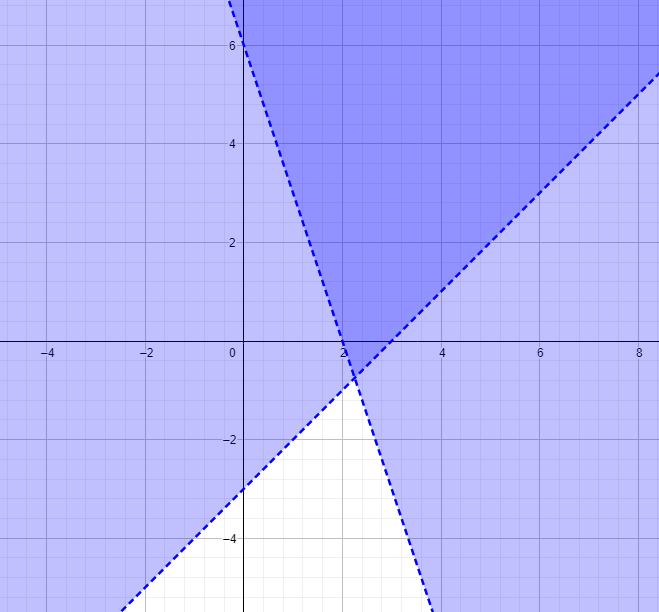# Algebra 1 - Systems of Equations and Inequalities

## Introduction

• A system of Linear Equations in two variables is generally written in the form of $\left\{\begin{array}{l}{a}_{1}x+{b}_{1}y={c}_{1}\\ {a}_{2}x+{b}_{2}y={c}_{2}\end{array}\right\$. This is also called the simultaneous linear equations in two variables.
• The pair of numbers (x,y) is called the solution that satisfies the equations in the system to become true.
• The following are some examples of linear systems in two variables: $\left\{\begin{array}{l}2x+y=8\\ 3x-y=7\end{array}\right\$$\left\{\begin{array}{l}y=x-9\\ 6x-6y=54\end{array}\right\$, and $\left\{\begin{array}{l}4x-y=5\\ 4x-y=1\end{array}\right\$.
• A single left-hand bracket typically signifies systems to replace the word "and."
• Two or more linear inequalities in two variables form a system of linear inequalities. A solution is an ordered pair that satisfies both inequalities in the system.
• A linear inequality in two variables is an inequality that can be expressed in any of the following forms:

## Types of Linear Systems in Two Variables

Consistent Equations (or Independent Equations):

• These are pairs of equations that have a unique solution. The point of intersection (x,y) is the solution of the given pair of equations.
• For any system of linear equations written in standard form $\left\{\begin{array}{l}{a}_{1}x+{b}_{1}y={c}_{1}\\ {a}_{2}x+{b}_{2}y={c}_{2}\end{array}\right\$, consistent equations have a form $\frac{{a}_{1}}{{a}_{2}}\ne \frac{{b}_{1}}{{b}_{2}}$.

Inconsistent Equations:

• These are sets of equations that have no solution. The intersection of two solution sets is an empty set.
• For any system of linear equations written in standard form $\left\{\begin{array}{l}{a}_{1}x+{b}_{1}y={c}_{1}\\ {a}_{2}x+{b}_{2}y={c}_{2}\end{array}\right\$, inconsistent equations have a form $\frac{{a}_{1}}{{a}_{2}}=\frac{{b}_{1}}{{b}_{2}}\ne \frac{{c}_{1}}{{c}_{2}}$.

Dependent Equations:

• These are equations in the system that have infinitely many solutions. These two linear equations are typically equivalent.
• For any system of linear equations written in standard form $\left\{\begin{array}{l}{a}_{1}x+{b}_{1}y={c}_{1}\\ {a}_{2}x+{b}_{2}y={c}_{2}\end{array}\right\$, dependent equations follow the form $\frac{{a}_{1}}{{a}_{2}}=\frac{{b}_{1}}{{b}_{2}}=\frac{{c}_{1}}{{c}_{2}}$

## Solved Examples Using Elimination and Substitution Method

Example 1. Solve the linear system $\left\{\begin{array}{l}2x-3y=-1\\ x+3y=4\end{array}\right\$ using the Elimination Method.

Solution:

Add two equations to eliminate y.

$\left(2x-3y=-1\right)+\left(x+3y=4\right)$

This forms $3x=3$ which yield $x=1$. Substitute the obtained value of x to any of the equations in the system to find the value of y.

$2\left(1\right)-3y=-1$

$2-3y=-1$

$-3y=-3$

$y=1$

Thus, the solutions to the system are .

Example 2. Solve the linear system $\left\{\begin{array}{l}3x+4y=2\\ y=x-3\end{array}\right\$ using the Substitution Method.

Solution:

Since we have $y=x-3$, substitute the expression $\left(x-3\right)$ in $3x+4y=2$ to solve for x.

$3x+4\left(x-3\right)=2$

$3x+4x-12=2$

$7x=14$

$x=2$

Now, substitute $x=2$ in $y=x-3$.

$y=2-3\to y=-1$

The solutions of the linear system are .

## Graphing Systems of Linear Equations

• To sketch the graph of the systems of linear equations, we have to locate the x-intercept and y-intercept. Graphs of a linear system in two variables determine the equations' type and solutions.
• The linear system is consistent and independent if the two lines intersect at one point.
• If the two lines are parallel, the linear system is inconsistent.
• If the equations have the same graph, the linear system is dependent.

## System of Linear Inequalities and their Graphs

• The graph of a linear inequality in two variables is a half-plane. The boundary line of the half-plane is dashed if the inequality is $<$ or $>$ and solid if the inequality is $\le$ or $\ge$.
• Graph each inequality in the system on the same set of axes.
• The graph of the solution set is the region common to the regions being graphed.
• Choose any arbitrary point within the solution set.
• Substitute the ordered pair to the original inequalities to check if it satisfies both relations.

## Solved Example of Linear Inequalities in Two Variables

Graph the system $\left\{\begin{array}{l}x-y<3\\ 3x+y>6\end{array}\right\$.

Solution:

Step 1. Sketch the graph of $x-y<3$. The graph is the open half-plane above the line $x-y=3$.

Step 2. Using the same coordinate grid, graph $3x+y>6$. The graph is the open half-plane above the line $3x+y=6$.

Notice that the graph of the system is the double-shaded area.Choose (2, 2) which is a point in the double-shaded region.

Substitute it to the inequalities in the system.

$x-y<3$

(TRUE)

$3x+y>6$

(TRUE)

## Cheat Sheet

• In the example $\left\{\begin{array}{l}2x+4y=-1\\ 4x+3y=5\end{array}\right\$, we multiply one or both equations in the system by a suitable number to find the solution. This is also a form of elimination method.
• If the slopes of the equations in the system are equal and the y-intercepts are not equal, then the linear system has no solution.
• If the slopes of the equations in the system are not equal and the y-intercepts are equal or unequal, the linear system has one solution.
• If both the slopes and y-intercepts of the equations in the system are equal, the linear system has infinite solutions.
• To determine whether the slopes of the lines in the system are equal, we can change the equations in slope-intercept form $\left\{\begin{array}{l}y={m}_{1}x+{b}_{1}\\ y={m}_{2}x+{b}_{2}\end{array}\right\$.
• In solving word problems involving linear systems in two variables or inequalities, read and understand the problem carefully. Decide which quantities are unknown and represent them in symbols. Based on the given conditions, formulate two linear models. Solve the system algebraically or graphically. Check your final answers based on the given conditions. For linear inequalities, sketching the graph is always required.

## Blunder Areas

• Always be mindful of using the basic integer operations when solving a linear system in two variables using algebraic methods.
• Not all systems of linear equations in two variables are written in standard form $\left\{\begin{array}{l}{a}_{1}x+{b}_{1}y={c}_{1}\\ {a}_{2}x+{b}_{2}y={c}_{2}\end{array}\right\$. In such cases, always simplify the equations in the system either in standard forms or slope-intercept forms.
• After finding the values of x and y, always check the values in both equations.
• Be careful with the use of strict/pure inequality symbols , and the not-pure inequality symbols when finding the solution of the linear system of inequalities in two variables.
• We can still use the elimination and substitution methods in solving a linear system of three variables. However, it is more convenient to use matrices and determinants.
• The two intersecting lines modeled by the equations in the linear system may be perpendicular. Inspect their slopes to see if ${m}_{1}=-\frac{1}{{m}_{2}}$.